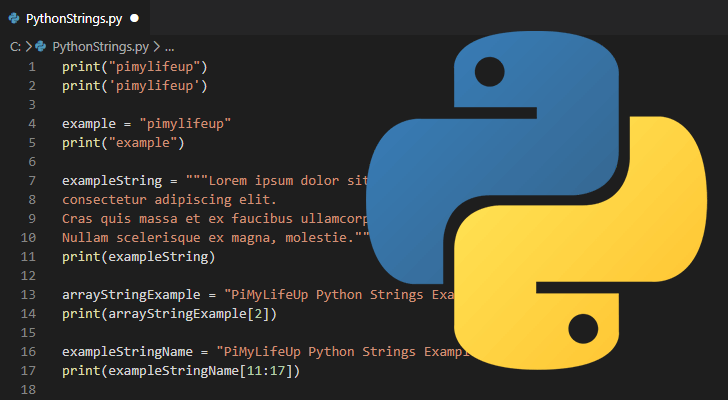# How to Pad String in Python

Python offers many features to work with numbers and strings. Sometimes you may need a string to be of specific length for processing it further. If your string is smaller than required length, you may need to pad the string with zeroes to increase its length. In this article, we will learn how to pad string in Python.

## How to Pad String in Python

Let us say you have the following string in python.

`n = '4'`

If you want to pad the above string to say 4 characters, then you can use zfill() function as shown below.

```>>> print(n.zfill(4))
0004```

## How to Pad Number in Python

On the other hand, if you have a number, not a string, then you can use any of the following print() commands with format() command to pad the number with zeroes to its left. It is just general string formatting.

```>>> n = 4
>>> print(f'{n:04}') # Preferred method, python >= 3.6
0004
>>> print('%04d' % n)
0004
>>> print(format(n, '04')) # python >= 2.6
0004
>>> print('{0:04d}'.format(n))  # python >= 2.6 + python 3
0004
>>> print('{foo:04d}'.format(foo=n))  # python >= 2.6 + python 3
0004
>>> print('{:04d}'.format(n))  # python >= 2.7 + python3
0004```

## How to Pad to Right in Python

The above methods pad strings & numbers to the left by prepending then with zeroes. If you want to pad the zeroes to right, you can use the following command.

```'{:<04d}'.format(n)
4000```

On the other hand, you can get left padding by slightly changing the format specifier in above command.

```'{:>04d}'.format(n)
0004```

In this article, we have learnt how to pad string and numbers with zeroes in Python. You can use any of these commands as per your requirement.